# Arithmetic genus

(diff) ← Older revision | Latest revision (diff) | Newer revision → (diff)

A numerical invariant of algebraic varieties (cf. Algebraic variety). For an arbitrary projective variety(over a field) all irreducible components of which have dimension, and which is defined by a homogeneous idealin the ring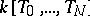, the arithmetic genus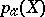is expressed using the constant term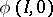of the Hilbert polynomialofby the formulaThis classical definition is due to F. Severi . In the general case it is equivalent to the following definition: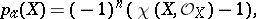whereis the Euler characteristic of the varietywith coefficients in the structure sheaf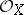. In this form the definition of the arithmetic genus can be applied to any complete algebraic variety, and this definition also shows the invariance ofrelative to biregular mappings. Ifis a non-singular connected variety, and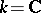is the field of complex numbers, then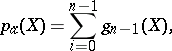where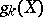is the dimension of the space of regular differential-forms on. Such a definition forwas given by the school of Italian geometers. For example, if, thenis the genus of the curve; if,whereis the irregularity of the surface, while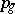is the geometric genus of.

For any divisoron a normal variety, O. Zariski (see ) defined the virtual arithmetic genus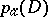as the constant term of the Hilbert polynomial of the coherent sheaf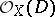corresponding to. If the divisorsand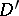are algebraically equivalent, one has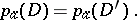The arithmetic genus is a birational invariant in the case of a fieldof characteristic zero; in the general case this has so far (1977) been proved for dimensions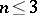only.

How to Cite This Entry:
Arithmetic genus. Encyclopedia of Mathematics. URL: http://encyclopediaofmath.org/index.php?title=Arithmetic_genus&oldid=14873
This article was adapted from an original article by I.V. Dolgachev (originator), which appeared in Encyclopedia of Mathematics - ISBN 1402006098. See original article